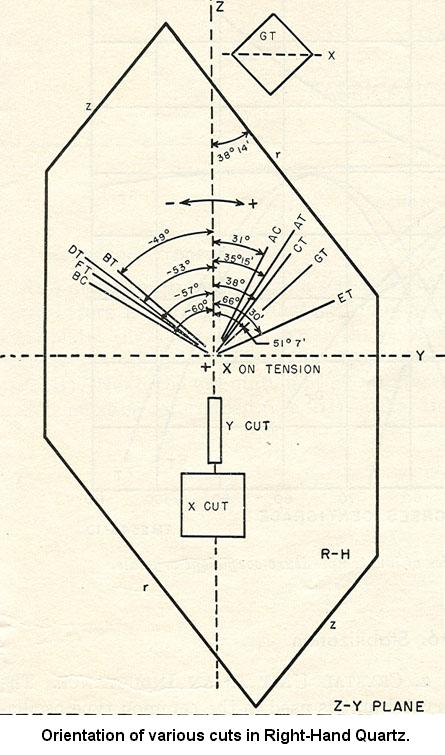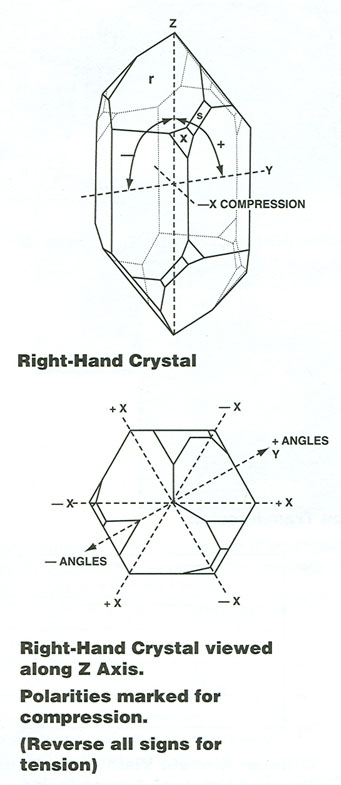## Crystal Quartz

Since approximately 1970 most crystal quartz components have been produced from man-made cultured quartz, which has the same physical, optical and electronic properties as natural quartz. Cultured quartz stones exhibit all the faceting of natural quartz except that the longest dimension is usually Y, whereas natural quartz is usually longest in Z.

### Material Characteristics

Physical Properties
Molecular weight 60.06
Density 2.65 g/cm3
Crystal class Hexagonal
Moh's hardness 7

Electrical Q

Mechanical Q

Approximately 2 million

180,000 - 290,000

Melting temperature 1610 °C
Curie point 573 °C
Thermal expansion

7.97 x 10-6/°C parallel to Z-axis in a temp. range from 0°C to 80°C.

13.37 x 10-6/°C perpendicular to Z-axis in a temp. range from 0°C to 80°C

Thermal conductivity @ 50 °C

0.0255 cal/(cm/sec/°C) parallel to Z-axis.

0.0148 cal/(cm/sec/°C) perpendicular to Z-axis.

Optical Properties
Refractive index (λ in µm)

no2 = 3.4269 + 1.0654 x 10-2 /C(λ2 - 0.010627)

ne2 = 3.5612557 + 0.00844614 /(λ2 - 0.0127493)

+ 0.00276113 /(λ2 - 0.000974)

+ 127.2 /(λ2 - 108)

201.9 °/mm @ 2265.03 Angstroms

95.02 °/mm @ 3034.12 Angstroms

21.724 °/mm @ 5892.9 Angstroms

11.589 °/mm @ 7947.63 Angstroms

0.972 °/mm @ 25000 Angstroms

Fresnel loss 8.2% @ 2 µm
Transmission range

0.4 µm - 4.5 µm

50 - 200 µm

### Piezoelectric & Dielectric Properties

 d11 = -2.30 x 10-12 C/N e11 = 0.171 C/m2 d14 = 0.67 e14 = 0.0403 g11 = -5.80 x 10-2 m2/C h11 = 4.36 x 109 N/C g14 = 1.82 x 10-2 h14 = 1.03 εs11/ε0 = 4.42 εT11/e0 = 4.52 εs33/ε0 = 4.63 εT33/ε0 = 4.70

### Elastic Constants

 Young's Modulus 14.9 PSI parallel to Z-axis 11.3 PSI perpendicular to Z-axis Modulus of Rigidity 6.65 PSI parallel to Z-axis 5.05 PSI perpendicular to Z-axis SE11 = 12.77 X 10-12 m2/N CE11 = 86.74 X 109 N/m2 SE12 = -1.79 CE12 = 6.99 SE13 = -1.22 CE13 = 11.91 SE14 = 4.50 CE14 = -17.91 SE33 = 9.60 CE33 = 107.2 SE44 = 20.04 CE44 = 57.94 SE66 = 29.12 CE66 = 39.88 SD11 = 12.79 CD11 = 87.49 SD12 = -1.54 CD12 = 6.87 SD13 = -1.10 CD13 = 11.91 SD14 = -4.46 CD14 = -18.09 SD33 = 9.56 CD33 = 107.2 SD44 = 19.78 CD44 = 57.98 SD66 = 28.66 CD66 = 40.63

### Direction Dependent Properties

Orientation X Y AT AC BC ST
Wave type Comp. Shear Shear Shear Shear Surface
Wave speed (m/s) 5700 3850 3320 3300 5000 3158
Coupling factor .10 -.14 -0.088 -.10 -.04 -.0011
d (pC/N) 2.3 -4.6 -3.4 -3.7 -0.9 ---